CGAL 5.2.1 - Poisson Surface Reconstruction
User Manual

# Introduction

This CGAL component implements a surface reconstruction method which takes as input point sets with oriented normals and computes an implicit function. We assume that the input points contain no outliers and little noise. The output surface mesh is generated by extracting an isosurface of this function with the CGAL Surface Mesh Generator  or potentially with any other surface contouring algorithm.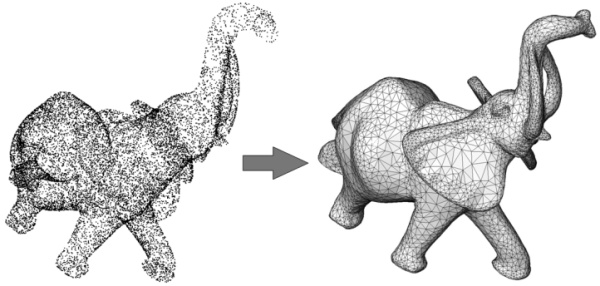Figure 60.1 Poisson surface reconstruction.
Left: 17K points sampled on the statue of an elephant with a Minolta laser scanner. Right: reconstructed surface mesh.

More specifically, the core surface reconstruction algorithm consists of computing an implicit function which is an approximate indicator function of the inferred solid (Poisson Surface Reconstruction - referred to as Poisson). Poisson is a two steps process: it requires solving for the implicit function before function evaluation.

Note
A detailed tutorial on surface reconstruction is provided with a guide to choose the most appropriate method along with pre- and post-processing.

# Common Reconstruction Pipeline

Surface reconstruction from point sets is often a sequential process with the following steps: 1) Scanning and scan alignment produce a set of points or points with normals; 2) Outlier removal; 3) Simplification to reduce the number of input points; 4) Smoothing to reduce noise in the input data; 5) Normal estimation and orientation when the normals are not already provided by the acquisition device; and 6) Surface reconstruction.

CGAL provides algorithms for all steps listed above except alignment.

Chapter Point Set Processing describes algorithms to pre-process the point set before reconstruction with functions devoted to the simplification, outlier removal, smoothing, normal estimation and normal orientation.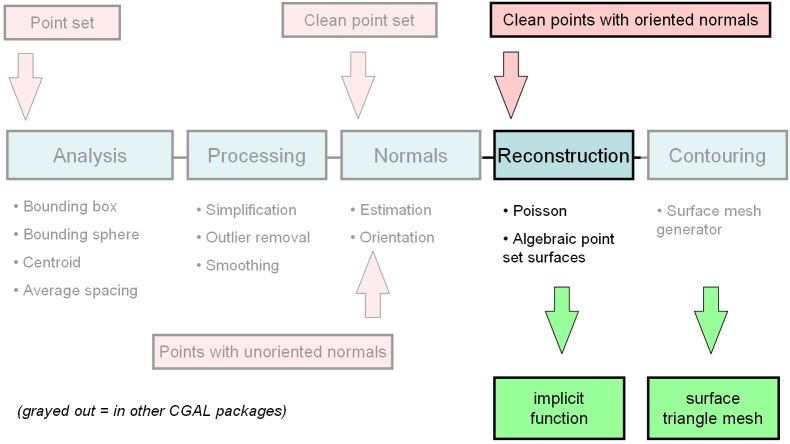Figure 60.2 Common surface reconstruction pipeline.

# Poisson

Given a set of 3D points with oriented normals (denoted oriented points in the sequel) sampled on the boundary of a 3D solid, the Poisson Surface Reconstruction method  solves for an approximate indicator function of the inferred solid, whose gradient best matches the input normals. The output scalar function, represented in an adaptive octree, is then iso-contoured using an adaptive marching cubes.

CGAL implements a variant of this algorithm which solves for a piecewise linear function on a 3D Delaunay triangulation instead of an adaptive octree. The algorithm takes as input a set of 3D oriented points. It builds a 3D Delaunay triangulation from these points and refines it by Delaunay refinement so as to remove all badly shaped (non isotropic) tetrahedra and to tessellate a loose bounding box of the input oriented points. The normal of each Steiner point added during refinement is set to zero. It then solves for a scalar indicator function $$f$$ represented as a piecewise linear function over the refined triangulation. More specifically, it solves for the Poisson equation $$\Delta f = div(\mathbf{n})$$ at each vertex of the triangulation using a sparse linear solver. Eventually, the CGAL surface mesh generator extracts an isosurface with function value set by default to be the median value of $$f$$ at all input points.

# Reconstruction Function

A global function poisson_surface_reconstruction_delaunay() is provided. It takes points with normals as input and handles the whole reconstruction pipeline :

• it computes the implicit function
• it reconstructs the surface with a given precision using the CGAL surface mesh generator based on Delaunay refinement  
• it outputs the result in a polygon mesh.

This function aims at providing a quick and user-friendly API for Poisson reconstruction. Advanced users may be interested in using the class (see Reconstruction Class) which allows them, for example, to use another surface mesher or a different output structure.

## Example

The following example reads a point set and reconstructs a surface using Poisson reconstruction.

#include <CGAL/Exact_predicates_inexact_constructions_kernel.h>
#include <CGAL/Polyhedron_3.h>
#include <CGAL/poisson_surface_reconstruction.h>
#include <CGAL/IO/read_xyz_points.h>
#include <vector>
#include <fstream>
// Types
typedef Kernel::Point_3 Point;
typedef Kernel::Vector_3 Vector;
typedef std::pair<Point, Vector> Pwn;
typedef CGAL::Polyhedron_3<Kernel> Polyhedron;
int main(void)
{
std::vector<Pwn> points;
std::ifstream stream("data/kitten.xyz");
if (!stream ||
stream,
std::back_inserter(points),
CGAL::parameters::point_map(CGAL::First_of_pair_property_map<Pwn>()).
normal_map(CGAL::Second_of_pair_property_map<Pwn>())))
{
std::cerr << "Error: cannot read file data/kitten.xyz" << std::endl;
return EXIT_FAILURE;
}
Polyhedron output_mesh;
double average_spacing = CGAL::compute_average_spacing<CGAL::Sequential_tag>
(points, 6, CGAL::parameters::point_map(CGAL::First_of_pair_property_map<Pwn>()));
(points.begin(), points.end(),
CGAL::First_of_pair_property_map<Pwn>(),
CGAL::Second_of_pair_property_map<Pwn>(),
output_mesh, average_spacing))
{
std::ofstream out("kitten_poisson-20-30-0.375.off");
out << output_mesh;
}
else
return EXIT_FAILURE;
return EXIT_SUCCESS;
}

# Reconstruction Class

The class template declaration is template<class Gt> class Poisson_reconstruction_function where Gt is a geometric traits class.

For details see: Poisson_reconstruction_function<GeomTraits>

## Example

The following example reads a point set, creates a Poisson implicit function and reconstructs a surface.

#include <CGAL/Exact_predicates_inexact_constructions_kernel.h>
#include <CGAL/Polyhedron_3.h>
#include <CGAL/Surface_mesh_default_triangulation_3.h>
#include <CGAL/make_surface_mesh.h>
#include <CGAL/Implicit_surface_3.h>
#include <CGAL/IO/facets_in_complex_2_to_triangle_mesh.h>
#include <CGAL/Poisson_reconstruction_function.h>
#include <CGAL/property_map.h>
#include <CGAL/IO/read_xyz_points.h>
#include <CGAL/compute_average_spacing.h>
#include <CGAL/Polygon_mesh_processing/distance.h>
#include <boost/iterator/transform_iterator.hpp>
#include <vector>
#include <fstream>
// Types
typedef Kernel::FT FT;
typedef Kernel::Point_3 Point;
typedef Kernel::Vector_3 Vector;
typedef std::pair<Point, Vector> Point_with_normal;
typedef CGAL::First_of_pair_property_map<Point_with_normal> Point_map;
typedef CGAL::Second_of_pair_property_map<Point_with_normal> Normal_map;
typedef Kernel::Sphere_3 Sphere;
typedef std::vector<Point_with_normal> PointList;
typedef CGAL::Polyhedron_3<Kernel> Polyhedron;
typedef CGAL::Poisson_reconstruction_function<Kernel> Poisson_reconstruction_function;
int main(void)
{
// Poisson options
FT sm_angle = 20.0; // Min triangle angle in degrees.
FT sm_radius = 30; // Max triangle size w.r.t. point set average spacing.
FT sm_distance = 0.375; // Surface Approximation error w.r.t. point set average spacing.
// Reads the point set file in points[].
// Note: read_xyz_points_and_normals() requires an iterator over points
// + property maps to access each point's position and normal.
PointList points;
std::ifstream stream("data/kitten.xyz");
if (!stream ||
stream,
std::back_inserter(points),
CGAL::parameters::point_map (Point_map()).
normal_map (Normal_map())))
{
std::cerr << "Error: cannot read file data/kitten.xyz" << std::endl;
return EXIT_FAILURE;
}
// Creates implicit function from the read points using the default solver.
// Note: this method requires an iterator over points
// + property maps to access each point's position and normal.
Poisson_reconstruction_function function(points.begin(), points.end(), Point_map(), Normal_map());
// Computes the Poisson indicator function f()
// at each vertex of the triangulation.
if ( ! function.compute_implicit_function() )
return EXIT_FAILURE;
// Computes average spacing
FT average_spacing = CGAL::compute_average_spacing<CGAL::Sequential_tag>
(points, 6 /* knn = 1 ring */,
CGAL::parameters::point_map (Point_map()));
// Gets one point inside the implicit surface
// and computes implicit function bounding sphere radius.
Point inner_point = function.get_inner_point();
Sphere bsphere = function.bounding_sphere();
FT radius = std::sqrt(bsphere.squared_radius());
// Defines the implicit surface: requires defining a
// conservative bounding sphere centered at inner point.
FT sm_sphere_radius = 5.0 * radius;
FT sm_dichotomy_error = sm_distance*average_spacing/1000.0; // Dichotomy error must be << sm_distance
Surface_3 surface(function,
Sphere(inner_point,sm_sphere_radius*sm_sphere_radius),
sm_dichotomy_error/sm_sphere_radius);
// Defines surface mesh generation criteria
CGAL::Surface_mesh_default_criteria_3<STr> criteria(sm_angle, // Min triangle angle (degrees)
sm_radius*average_spacing, // Max triangle size
sm_distance*average_spacing); // Approximation error
// Generates surface mesh with manifold option
STr tr; // 3D Delaunay triangulation for surface mesh generation
C2t3 c2t3(tr); // 2D complex in 3D Delaunay triangulation
CGAL::make_surface_mesh(c2t3, // reconstructed mesh
surface, // implicit surface
criteria, // meshing criteria
CGAL::Manifold_with_boundary_tag()); // require manifold mesh
if(tr.number_of_vertices() == 0)
return EXIT_FAILURE;
// saves reconstructed surface mesh
std::ofstream out("kitten_poisson-20-30-0.375.off");
Polyhedron output_mesh;
out << output_mesh;
// computes the approximation error of the reconstruction
double max_dist =
CGAL::Polygon_mesh_processing::approximate_max_distance_to_point_set
(output_mesh,
CGAL::make_range (boost::make_transform_iterator
(points.begin(), CGAL::Property_map_to_unary_function<Point_map>()),
boost::make_transform_iterator
(points.end(), CGAL::Property_map_to_unary_function<Point_map>())),
4000);
std::cout << "Max distance to point_set: " << max_dist << std::endl;
return EXIT_SUCCESS;
}

## Contouring

The computed implicit functions can be iso-contoured to reconstruct a surface by using the CGAL surface mesh generator   :

make_surface_mesh()

The parameter Tag affects the behavior of make_surface_mesh():

• Manifold_tag: the output mesh is guaranteed to be a manifold surface without boundary.
• Manifold_with_boundary_tag: the output mesh is guaranteed to be manifold and may have boundaries.
• Non_manifold_tag: the output mesh has no guarantee and hence is outputted as a polygon soup.

## Output

The surface reconstructed by make_surface_mesh() is required to be a model of the concept SurfaceMeshComplex_2InTriangulation_3, a data structure devised to represent a two dimensional complex embedded into a three dimensional triangulation.

SurfaceMeshComplex_2InTriangulation_3 defines the methods to traverse the reconstructed surface, and e.g. convert it to a triangle soup.

Other CGAL components provide functions to write the reconstructed surface mesh to the Object File Format (OFF)  and to convert it to a polyhedron (when it is manifold):

• output_surface_facets_to_off()
• output_surface_facets_to_polyhedron()

See poisson_reconstruction_example.cpp example above.

# Case Studies

The surface reconstruction problem being inherently ill-posed, the proposed algorithm does not pretend to reconstruct all kinds of surfaces with arbitrary sampling conditions. This section provides the user with some hints about the ideal sampling and contouring conditions, and depicts some failure cases when these conditions are not matched.

## Ideal Conditions

The user must keep in mind that the Poisson surface reconstruction algorithm comprises two phases (computing the implicit function from the input point set and contouring an iso-surface of this function). Both require some care in terms of sampling conditions and parameter tuning.

## Point Set

Ideally the current implementation of the Poisson surface reconstruction method expects a dense 3D oriented point set (typically matching the epsilon-sampling condition ) and sampled over a closed, smooth surface. Oriented herein means that all 3D points must come with consistently oriented normals to the inferred surface. Figure 60.3 and Figure 60.4 illustrate cases where these ideal conditions are met.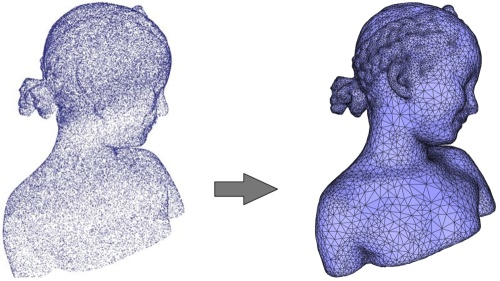Figure 60.3 Poisson reconstruction. Left: 120K points sampled on a statue (Minolta laser scanner). Right: reconstructed surface mesh.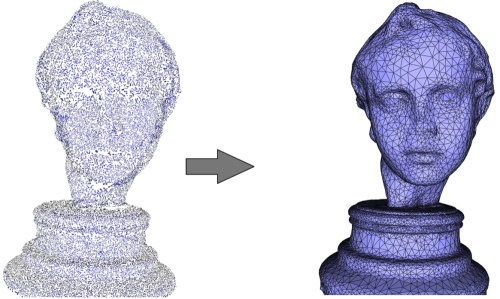Figure 60.4 Left: 120K points sampled on a statue (Minolta laser scanner). Right: reconstructed surface mesh.

The algorithm is fairly robust to anisotropic sampling and to noise. It is also robust to missing data through filling the corresponding holes as the algorithm is designed to reconstruct the indicator function of an inferred solid (see Figure 60.5).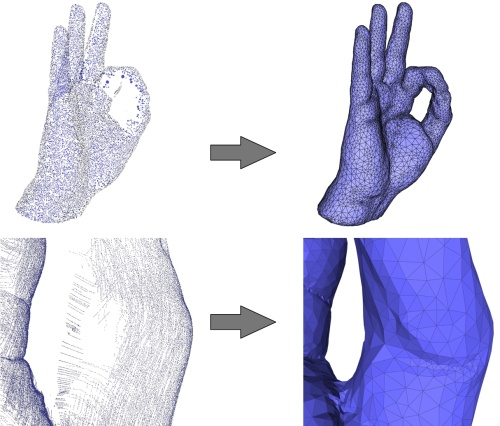Figure 60.5 Top left: 65K points sampled on a hand (Kreon laser scanner). Bottom left: the point set is highly anisotropic due to the scanning technology. Right: reconstructed surface mesh and closeup. The holes are properly closed.

The algorithm is in general not robust to outliers, although a few outliers do not always create a failure, see Figure 60.6.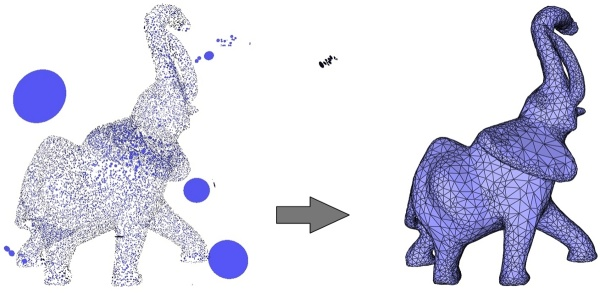Figure 60.6 Left: 70K points sampled on an elephant with few outliers emphasized with disks. Right: reconstructed surface mesh.

The algorithm works well even when the inferred surface is composed of several connected components, provided that both all normals are properly estimated and oriented (the current CGAL normal orienter algorithm may fail in some cases, see mst_orient_normals()), and that the final contouring algorithm is properly seeded for each component. When the inferred surface is composed of several nested connected components care should be taken to orient the normals of each component in alternation (inward/outward) so that the final contouring stage picks a proper contouring value.

## Contouring Parameters

Our implementation of the Poisson surface reconstruction algorithm computes an implicit function represented as a piecewise linear function over the tetrahedra of a 3D Delaunay triangulation constructed from the input points then refined through Delaunay refinement. For this reason, any iso-surface is also piecewise linear and hence may contain sharp creases. As the contouring algorithm make_surface_mesh() expects a smooth implicit function these sharp creases may create spurious clusters of vertices in the final reconstructed surface mesh when setting a small mesh sizing or surface approximation error parameter (see Figure 60.7).

One way to avoid these spurious clusters consists of adjusting the mesh sizing and surface approximation parameters large enough compared to the average sampling density (obtained through compute_average_spacing()) so that the contouring algorithm perceives a smooth iso-surface. We recommend to use the following contouring parameters:

• Max triangle radius: at least 100 times the average spacing.
• Approximation distance: at least 0.25 times the average spacing.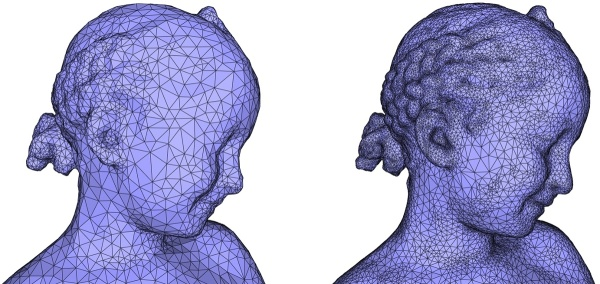Figure 60.7 Left: surface reconstructed with approximation distance = 0.25 * average spacing. Right: surface reconstructed with approximation distance = 0.15 * average spacing. Notice the spurious cluster on the cheek.

## Degraded Conditions

The conditions listed above are rather restrictive and in practice not all of them are met in the applications. We now illustrates the behavior of the algorithm when the conditions are not met in terms of sampling, wrongly oriented normals, noise and sharp creases.

## Sparse Sampling

The reconstruction algorithm expects a sufficiently dense point set. Although there is no formal proof of correctness of the algorithm under certain density conditions due to its variational nature, our experiments show that the algorithm reconstructs well all thin features when the local spacing is at most one tenth of the local feature size (the distance to the medial axis, which captures altogether curvature, thickness and separation). When this condition is not met the reconstruction does not reconstruct the thin undersampled features (see Figure 60.8).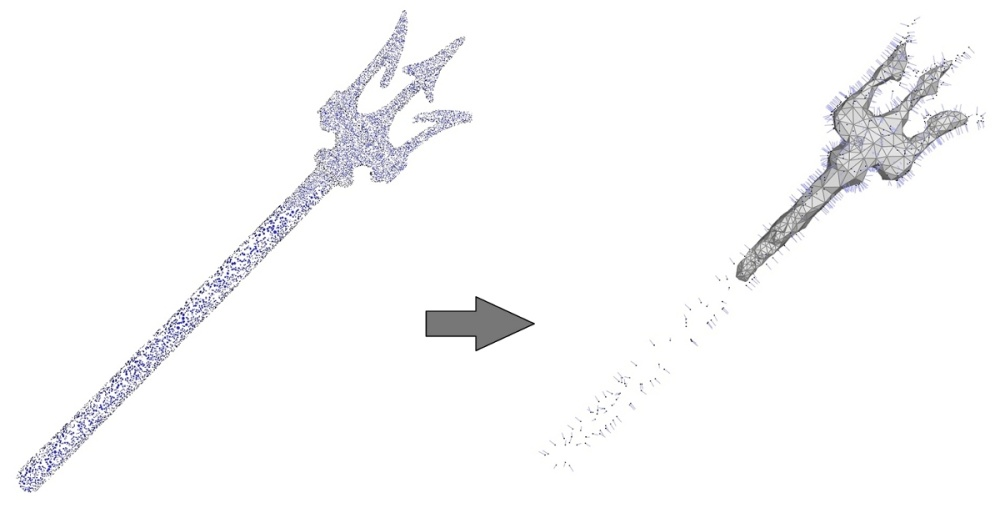Figure 60.8 Left: 50K points sampled on the Neptune trident. The reconstruction (not shown) is successful in this case. Right: point set simplified to 1K points then reconstructed (all input points are depicted with normals). The thin feature is not reconstructed.

## Large Holes

The reconstruction is devised to solve for an implicit function which is an approximate indicator function of an inferred solid. For this reason the contouring algorithm always extracts a closed surface mesh and hence is able to fill the small holes where data are missing due, e.g., to occlusions during acquisition (see Figure 60.9).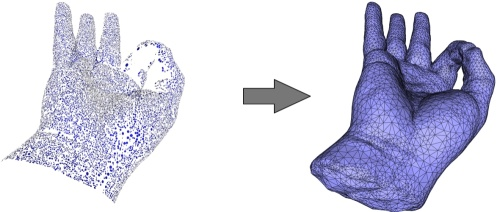Figure 60.9 Left: 65K points sampled on a hand with no data captured at the wrist base. Right: reconstructed surface mesh. The surface is properly closed on the fingers and also closed at the wrist but in a less plausible manner.

In case of large holes the algorithm still closes them all but the resulting piecewise linear implicit function may exhibit large triangle patches and sharp creases as the 3D Delaunay triangulation used for solving is very coarse where the holes are filled. This can be avoided by a two pass approach. The first pass for a subset of the points serves to get an approximation of the surface at the holes. This surface then serves to compute a smoother 3D Delaunay triangulation for the second pass with the full set of points.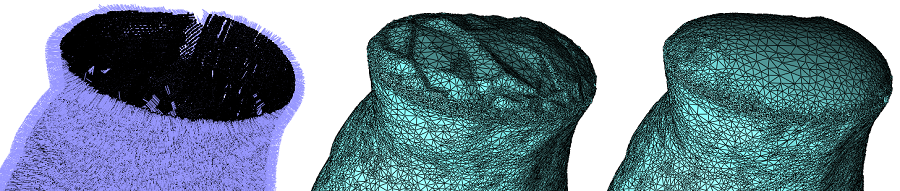Figure 60.10 Left: The wrist. Middle: one pass. Right: two passes.

## Wrongly Oriented Normals

The Poisson surface reconstruction approaches solves for an implicit function whose gradient best matches a set of input normals. Because it solves this problem in the least squares sense, it is robust to few isolated wrongly oriented (flipped) normals. Nevertheless a cluster of wrongly oriented normals leads to an incorrect implicit function and hence to spurious geometric or even topological distortion (see Figure 60.11).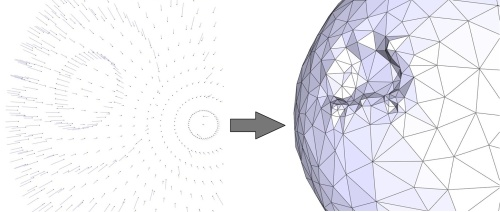Figure 60.11 Left: points sampled on a sphere with a cluster of wrongly oriented normals. Right: reconstructed surface mesh with a spurious bump.

## Noise and Outliers

A large amount of noise inevitably impacts on the reconstruction (see Figure 60.12, top) and the current implementation does not provide any mean to trade data fitting for smoothness. Nevertheless if the signal-to-noise ratio is sufficiently high and/or the surface approximation and sizing parameters set for contouring the iso-surface is large with respect to the noise level the output surface mesh will appear smooth (not shown). If the user wants to produce a smooth and detailed output surface mesh, we recommend to apply smoothing through jet_smooth_point_set() (see Figure 60.12, bottom).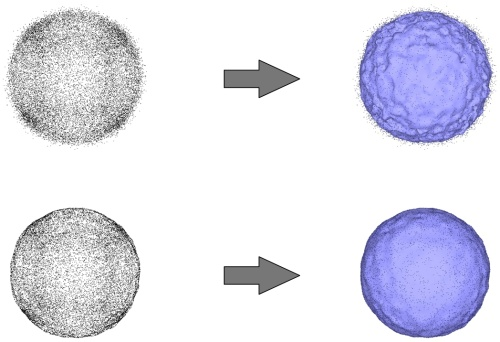Figure 60.12 Top-left: points sampled on a sphere and corrupted with a lot of noise. Top-right: reconstructed surface mesh. Bottom-left: smoothed point set. Bottom-right: reconstructed surface mesh.

For a large number of outliers the failure cases (not shown) translate into spurious small connected components and massive distortion near the inferred surface. In this case the outliers must be removed through remove_outliers().

## Sharp Creases

The current reconstruction algorithm is not able to recover the sharp creases and corners present in the inferred surface. This translates into smoothed sharp creases.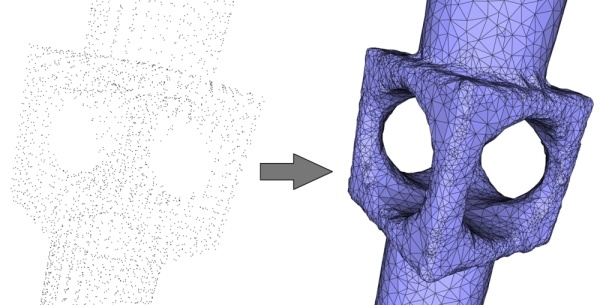Figure 60.13 Left: 5K points sampled on a mechanical piece with sharp features (creases, darts and corners). Right: reconstructed surface mesh with smoothed creases.

# Performances

We provide some performance numbers for scanning data. We measure the Poisson implicit function computation time, the contouring time for a range of approximation distances, the memory occupancy as well as the influence of the point set simplification. The machine used is a PC running Windows 7 64 bits with an Intel CPU Core 2 Duo processor clocked at 2.81 GHz and with 8 GB of RAM. The software is compiled with Visual C++ 2010 (VC9) compiler with the 03 option which maximizes speed. All measurements were done using the Eigen library.

## Poisson Implicit Function

The point set chosen for benchmarking the Poisson implicit function is the Bimba con Nastrino point set (1.6 million points) depicted by Figure 60.14. We measure the Poisson implicit function computation (i.e., the call to Poisson_reconstruction_function::compute_implicit_function() denoted by Poisson solve hereafter) for this point set as well as for simplified versions obtained through random simplification. The following table provides Poisson solve computation times in seconds for an increasing number of points.

 Number of points (x1000) Poisson solve duration (in s) 60 15 100 25 250 96 500 150 1,000 249 1,800 478

## Contouring

The point set chosen for benchmarking the contouring stage is the Bimba con Nastrino point set simplified to 100k points. We measure the contouring (i.e. the call to make_surface_mesh()) duration and the reconstruction error for a range of approximation distances. The reconstruction error is expressed as the average distance from input points to the reconstructed surface in mm (the Bimba con Nastrino statue is 324 mm tall).

 Approx. distance (*average spacing) Contouring duration (in s) Reconstruction error (mm) 0.1 19.2 0.055 0.25 6.9 0.106 0.5 3.2 0.18 1 1.65 0.36 2 0.8 0.76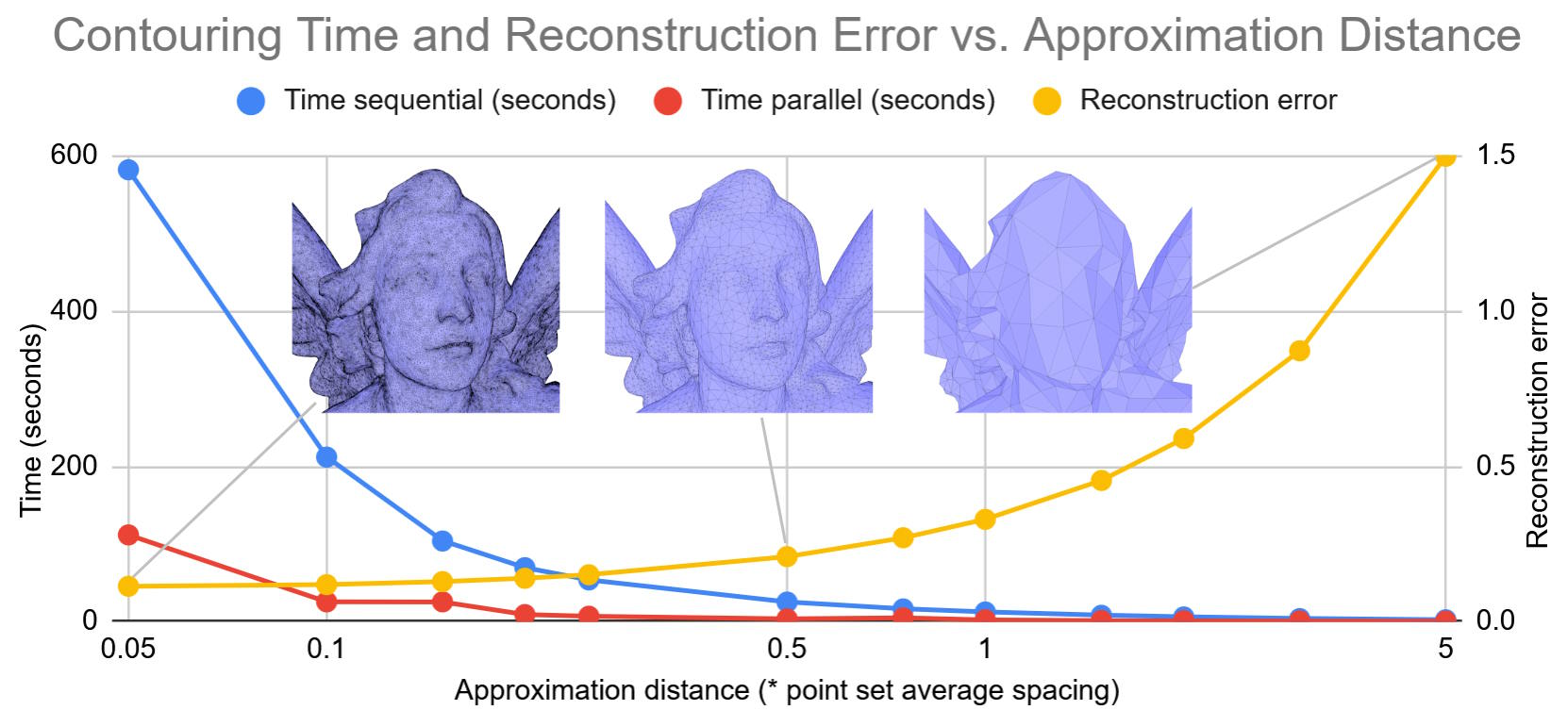Figure 60.14 Contouring duration (in s) and reconstruction error (mm) against several approximation distance parameters for the Bimba con Nastrino point set simplified to 100k points.

## Memory

We measure the memory occupancy for the reconstruction of the full Bimba con Nastrino point set (1.8 millions points) as well as for simplified versions.
The Poisson implicit function computation has a memory peak when solving the Poisson linear system using the sparse linear solver.

 Number of points (x1000) Memory occupancy (MBytes) 60 180 100 270 250 790 500 1300 1,000 2200 1,800 3800

## Point Set Simplification

Due to the memory limitations described above, we recommend to simplify the point sets captured by laser scanners.
We measure the reconstruction error for the Bimba con Nastrino point set (1.6M points) as well as for simplified versions. All reconstructions use the recommended contouring parameter approximation distance = 0.25 * the input point set's average spacing. The reconstruction error is expressed as the average distance from input points to the reconstructed surface in mm (the Bimba con Nastrino statue is 324 mm tall).

 Number of points (x1000) Reconstruction error (mm) 60 0.27 120 0.15 250 0.11 500 0.079 1,000 0.066 1,500 0.061 1,600 0.06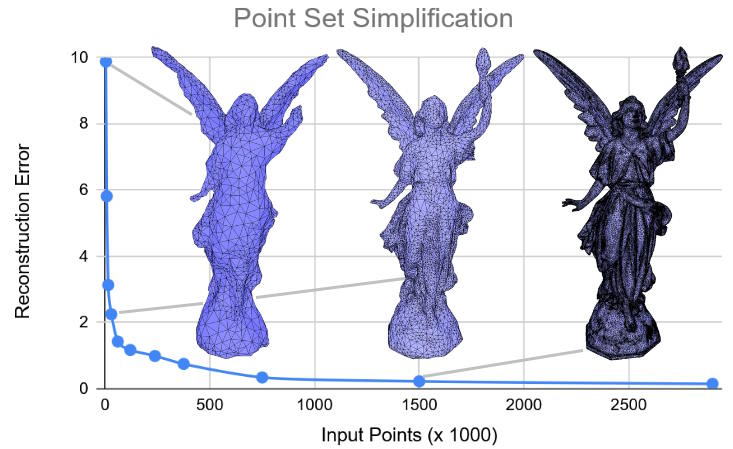Figure 60.15 Reconstruction error (mm) against number of points for the Bimba con Nastrino point set with 1.6M points as well as for simplified versions.

# Design and Implementation History

The initial implementation was essentially done by Laurent Saboret, guided by Pierre Alliez and Ga"el Guennebaud. For later releases of the package Andreas Fabri worked on performance improvements, and Laurent Rineau added the two passes for dealing with holes.